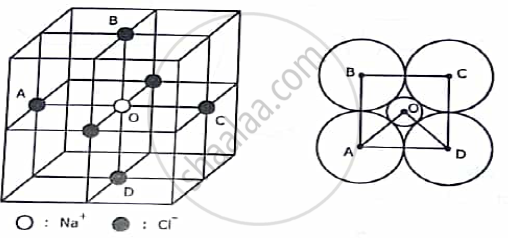Advertisement Remove all ads

# Define ligancy and critical radius ratio. Calculate critical radius radio for ligancy 6. - Applied Physics 1

Short Note

Define ligancy and critical radius ratio. Calculate critical radius radio for ligancy 6.

Advertisement Remove all ads

#### Solution

LIGANCY:-
In an ionic solid the cation and anion are positioned at alternate lattice points. Generally cations are smaller than anions in size. In a given crystal the number of anions surrounding a cation is called the ligancy i.e., the coordination number of an ionic crystal.
RADIUS RATIO:-
If the surrounding anions touch each other as well as touch the central cations the condition is called critical. In this case the cation-anion radius ratio is called the critical radius ratio rC /rA . Here rC and rA are the cation and anion radii respectively.
OCTAHEDRAL CONFIGURATION:
The octahedral configuration of neighbouring anions is found in NaCl structure. Here four anions A, B ,C and D are arranged at the corners of a square with the cation O at the centre of the square. Two more anions are situated in front and at the back of the cation. The centres of all six anions form an octahedron.

Here in ∆ BOC , < BOC = 90°, BC = 2r , OB = rC + rA and <BCO =45°,

Hence, ( BO)/(BC) = cos45°

Or, ( r_C +r _A)/(2r_A) = 1/(√2)The critical radius ratio here is,

(r_C)/(r_A)=0.414

Concept: Study of characteristics of unit cell of Diamond, ZnS, NaCl and HCP;
Is there an error in this question or solution?
Advertisement Remove all ads

#### APPEARS IN

Advertisement Remove all ads
Advertisement Remove all ads
Share
Notifications

View all notifications

Forgot password?